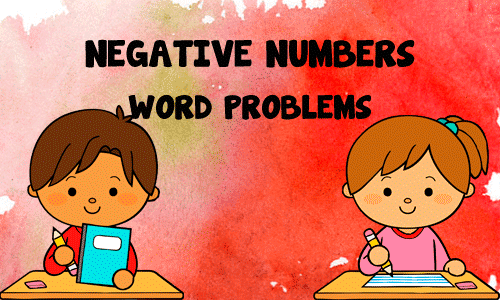Integers Word Problems

13 QuestionsSettingsPositive and negative numbers word problems. Solve the following problems by adding or subtracting the given amounts.

• 1.
The Tunnel of Fear ride climbs straight up to its peak 50 meters above the ground. Then, it drops 65 meters into an underground tunnel. What is the height relative to the ground?
• A.

-15

• B.

15

• C.

115

• D.

-115

• 2.
The temperature on Tuesday is 5°C.  On Wednesday it is 6 degrees colder.  What is the temperature on Wednesday?
• A.

-1ºC

• B.

1ºC

• C.

11ºC

• D.

-11ºC

• 3.
The temperature on Friday is -7°C.  The following the day, the temperature rises by 9 degrees.  What is the temperature the following day?
• A.

2ºC

• B.

-2ºC

• C.

16ºC

• D.

-16ºC

• 4.
The temperature in London is -5°C.  In Birmingham it is -8°C.  What is the difference between the 2 temperatures?
• A.

-3ºC

• B.

-5ºC

• C.

-13ºC

• D.

5ºC

• 5.
How many degrees more is 2°C than -2°C?
• A.

4ºC

• B.

-4ºC

• C.

2ºC

• D.

-8ºC

• 6.
The temperature on Sunday is 4°C.  The following day it drops by 11°C.  What is the temperature on the following day?
• A.

-7ºC

• B.

15ºC

• C.

-15ºC

• D.

7ºC

• 7.
The temperature is -9°C.  If it is 8°C warmer on the following day, what will the temperature be?
• A.

-1ºC

• B.

1ºC

• C.

17ºC

• D.

-17ºC

• 8.
The temperature is -6°C.  The following day it is 9 degrees warmer.  What is the temperature on the following day?
• A.

3ºC

• B.

-3ºC

• C.

15ºC

• D.

23ºC

• 9.
In November the temperature was 18°C.  During the night the temperature dropped by 20 degrees.  What was the temperature during the night?
• A.

-2ºC

• B.

2ºC

• C.

18ºC

• D.

38ºC

• 10.
A skyscraper has floors below as well as above ground.  If I start at the fifth floor and go down eight floors how many floors below ground level will I be?
• A.

There will be 3 floors underground.

• B.

-4

• C.

There will be 4 floors underground.

• D.

There will be 2 floors underground.

• 11.
One day in December the temperature was 4°C.  During the night the temperature dropped to -5°C.  How many degrees colder was it is the night than in the day?
• A.

It was 9 degrees colder.

• B.

It was 18 degrees colder.

• C.

It was 12 degrees colder.

• D.

It was 1 degree colder.

• 12.
A skyscraper has floors below as well as many above ground.  If I start at the 35th floor and go down 40 floors how many floors below ground level will I be?
• A.

I will be at floor -5.

• B.

I will be at floor 75.

• C.

I will be at floor -35.

• D.

I will be at the Ministry of Magic.

• 13.
In January the temperature one day was -3°C.  In the night time the temperature dropped another 4 degrees.  What was the temperature at night?
• A.

-7ºC

• B.

7ºC

• C.

-1ºC

• D.

1ºC